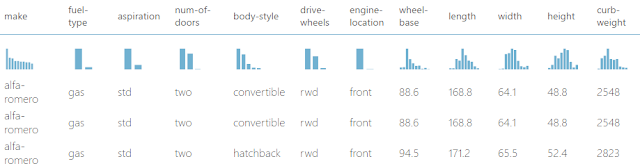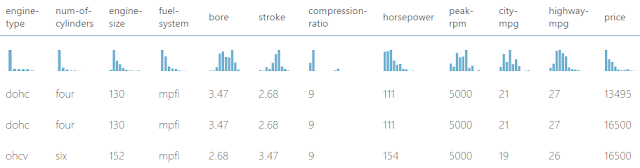## Monday, March 20, 2017

### Azure Machine Learning: Regression Using Linear Regression (Ordinary Least Squares)

Today, we're going to continue our walkthrough of Sample 4: Cross Validation for Regression: Auto Imports Dataset.  In the previous post, we walked through the initial data load and imputation phases of the experiment.Initial Data Load and Imputation
Let's refresh our memory on the data set.Automobile Price Data (Clean) 1Automobile Price Data (Clean) 2
We can see that this data set contains a bunch of text and numeric data about each vehicle, as well as its price.  The goal of this experiment is to attempt to predict the price of the car based on these factors.  One way to do this is through a technique called Regression.  Basically, regression is a technique for predicting a numeric value (or set of values) based on a series of numeric inputs.

Now, some of you might be asking what happens to the non-numeric text data.  Turns out, they get converted into numeric variables using a technique called Indicator Variables (also known as Dummy Variables).  With this technique, every text field gets broken down into multiple binary (0/1) fields, each representing a single unique value from the original field.  For instance, the Num of Doors fields takes the values "two" and "four".  Therefore, the Indicator Variables for this field would be "Num of Doors = two" and "Num of Doors = four".  Each of these fields takes a value of 1 if the original field contains the value in question, and 0 if it doesn't.  To continue our example, a vehicle with "Num of Doors" = "two" would have a value of 1 in the "Num of Doors = two" field and a value of 0 in the "Num of Doors = four" field.Indicator Variables Example
Things actually get a little more complicated when you are dealing with Unknown/NULL values.  The specific technique used varies based on the tool, but rarely has any effect.  We'll see how things works for Linear Regression later in this post.  As a side note, not all modules will automatically convert your fields to Indicator Variables.  In these cases, Azure ML has a module called Convert to Indicator Values that will do this for you.  If we need finer control over exactly how it accomplishes this, we could also use a SQL, R or Python script to handle it.  Let's move on to Linear Regression.

Earlier, we mentioned that Regression is a technique for predicting numeric values using other numeric values.  Linear Regression is a subset of Regression that creates a very specific type of model.  Let's say that we are trying to predict a value x by using values y and z.  A linear regression algorithm will create a model that looks like x = a*y + b*z + c, where a, b and c are called "coefficients", also known as "weights".  Now, this relationship looks linear from the coefficients' perspectives (meaning that there are no exponents, trigonometric functions, etc.).  However, if were to alter our data set so that z = y^2, then we would end up with the model x = a*y + b*y^2 + c.  This is LINEAR from the coefficients' perspectives, but is PARABOLIC from variables' perspectives.  This is one of the major reasons why Linear Regression is so popular.  It's very easy to build, train and comprehend, but is virtually limitless in the amount of relationships it can handle.  Let's take a look at the parameters.Linear Regression (OLS)
We see that there are two options for "Solution Method".  The first, and most common, method is "Ordinary Least Squares" (OLS).  This method is the one most commonly taught because it has almost no parameters to tinker with.  We basically toss our data at it and it runs.  OLS is also very efficient because the entire algorithm is just a short series of linear algebra operations and only runs through the data once.  You can learn more about OLS here.

The second option for "Solution Method" is "Online Gradient Descent".  This method is substantially more complicated and will be covered in the next post.

Without going into too much depth, the "L2 Regularization Weight" parameter penalizes complex models.  Unfortunately, the "Tune Model Hyperparameters" module will not choose this value for us.  On the other hand, we tried a few values and did not find it to have any significant impact on our model.  If you want to learn more about Regularization, read this and this.

We can also choose "Include Intercept Term".  If we deselect this option, then our model will change from x = a*y + b*z + c to x = a*y + b*z.  This means that when all of our factors are 0, then our prediction would also be zero.  Honestly, we've never found a reason, in school or in practice, why we would ever want to deselect this option.  If you know of any, please let us know in the comments.

Next, we can choose a "Random Number Seed".  Most machine learning algorithms are random by nature.  That means their "starting point" matters.  Running the algorithm multiple times will produce different results.  However, the OLS algorithm is not random.  We tested and confirmed that this parameter has no impact on this algorithm.

Finally, we can choose to deselect "Allow Unknown Categorical Levels".  When we train our model, we do so using a specific data set known as the training set.  This allows the model to predict based on values it has seen before.  For instance, our model has seen "Num of Doors" values of "two" and "four".  So, what happens if we try to use the model to predict the price for a vehicle with a "Num of Doors" value of "three" or "five"?  If we leave this option selected, then this new vehicle will have its "Num of Doors" value thrown into an "Unknown" category.  This would mean that if we had a vehicle with three doors and another vehicle with five doors, they would both be thrown into the same "Num of Doors" category.  We'll see exactly how this works when we look at the indicator variables.

Now that we know understand the parameters behind the OLS module, let's look at the results of the "Train Model" module.Train ModelTrain Model (Visualization)

We can see that the visualization is made up of two sections, "Settings" and "Feature Weights".  The "Settings" section simply shows us what parameters we set in the module.  The "Feature Weights" section shows us all of the independent variables (everything except what we were trying to predict, which was Price) as well as their "Weight" or "Coefficient".  Positive weights mean that the value has a positive effect on price and Negative weights mean that the value has a negative effect on price.  Let's take a closer look at some of the different features.Features
We can see that there are quite a few different features in this model.  We've pulled out a few and color coded them for clarity.  Let's start with "Bias".  Remember back to our model equation, x = a*y + b*z + c.  The "Bias" value corresponds to c in our equation.  This tells us that if all of our other factors were 0 (which is impossible for some of our factors), the price of our car would be -\$6,008.57.  Obviously, this is a silly value.  Bias, also known as the intercept, is not generally a useful value by itself.  However, it does greatly improve the fit of our models and can be utilized by more advanced techniques.

Next, let's take a look at the features in Grey.  These are all numeric features.  We can tell because they don't have any underscores (_) or pound signs (#) in them.  We see that cars with an additional "Width" of 1, would also have an additional price of \$600.89.  We can also see that vehicles will larger values of "Bore" and "Stroke" have lower prices.

Let's move on to the features in Blue.  These are the Indicator Variables we've mentioned a couple of time.  In our original data set, we included a feature called "body-style".  This feature had the values "convertible", "hardtop", "hatchback", "sedan" and "wagon".  Therefore, when the Linear Regression module needed to convert these to Indicator Variables, it used an extremely simple method.  It created new fields with the titles of "<field name>_<field value>_<index>".  The <field name> and <field value> are pulled directly from the record, while <index> is created by ordering the values (notice how they are in alphabetical order?) and counting up from 0.

Now, since we didn't deselect the "Allow Unknown Categorical Levels" option, we have an additional feature for each text field.  This field is named "<field name>#unknown_<index>".  This is the additional category that any new values from the testing set would be thrown into.  Currently, we're not quite sure how it assigns a weight to a value it hasn't seen.  If you know, please let us know in the comments.  It's also interesting to note that the index for the unknown category is not calculated correctly.  It appears to be calculated as [Number of Values] + 1.  However, since indexes start counting at 0 instead of 1, our index is always one larger than it should be.  For instance, the indexes for the "num-of-doors" fields are 0, 1, 2 and 4.

Finally, let's take a look at the "num-of-doors" fields in Purple.  In the previous post <INSERT LINK HERE>, we had some missing values in the "num-of-doors" field.  These values were replaced with a value of "Unknown".  Since "Unknown" is a valid value in our data set, we end up with two different unknown fields in our final result, "num-of-doors_unknown_2" (defined by us) and "num-of-doors#unknown_4" (defined by the algorithm).  This isn't significant; it's just interesting.

As a final note, if we were to perform Linear Regression in other tools, we would be able to access a summary table telling us whether each individual variable was "statistically significant".  For instance, here's a sample R output we pulled from Google.

``````Call:
lm(formula = a1 ~ ., data = clean.algae[, 1:12])

Residuals:
Min      1Q  Median      3Q     Max
-37.679 -11.893  -2.567   7.410  62.190

Coefficients:
Estimate Std. Error t value Pr(>|t|)
(Intercept)  42.942055  24.010879   1.788  0.07537 .
seasonspring  3.726978   4.137741   0.901  0.36892
seasonsummer  0.747597   4.020711   0.186  0.85270
seasonwinter  3.692955   3.865391   0.955  0.34065
sizemedium    3.263728   3.802051   0.858  0.39179
sizesmall     9.682140   4.179971   2.316  0.02166 *
speedlow      3.922084   4.706315   0.833  0.40573
speedmedium   0.246764   3.241874   0.076  0.93941
mxPH         -3.589118   2.703528  -1.328  0.18598
mnO2          1.052636   0.705018   1.493  0.13715
Cl           -0.040172   0.033661  -1.193  0.23426
NO3          -1.511235   0.551339  -2.741  0.00674 **
NH4           0.001634   0.001003   1.628  0.10516
oPO4         -0.005435   0.039884  -0.136  0.89177
PO4          -0.052241   0.030755  -1.699  0.09109 .
Chla         -0.088022   0.079998  -1.100  0.27265
---
Signif. codes:  0 ‘***’ 0.001 ‘**’ 0.01 ‘*’ 0.05 ‘.’ 0.1 ‘ ’ 1

Residual standard error: 17.65 on 182 degrees of freedom
Multiple R-squared:  0.3731,    Adjusted R-squared:  0.3215  ``````
F-statistic: 7.223 on 15 and 182 DF, p-value: 2.444e-12

Using this table, we can find out which variables are useful and which are not.  Unfortunately, we were not able to find a way to create this table using any of the built-in modules.  We could certainly use and R or Python script to do it, but that's beyond the scope of this post.  Once again, if you have any insight, please share it with us.

Hopefully, this post enlightened you to the possibilities of OLS Linear Regression.  It truly is one of the easiest, yet most powerful techniques in all of Data Science.  It's made even easier by its use in Azure Machine Learning Studio.  Stay tuned for the next post where we'll dig into the other type of Linear Regression, Online Gradient Descent.  Thanks for reading.  We hope you found this informative.

Brad Llewellyn
Data Scientist
Valorem
@BreakingBI
www.linkedin.com/in/bradllewellyn
llewellyn.wb@gmail.com

#### 2 comments:

1.I agree that it's frustrating about the p-values not being exposed or use-able during the discovery process, as this is my primary method of feature selecting...

Appreciate the post!

2.Thanks! Good info here.